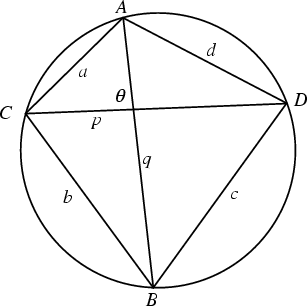A Quadrilateral for which a Circle can be circumscribed so that it touches each Vertex. The Area is then given by a special case of Bretschneider's Formula. Let the sides have lengths,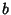,, and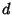, let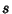be the Semiperimeter(1)

and let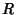be the Circumradius. Then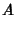(2)(3)(4)

The Diagonals of a cyclic quadrilateral have lengths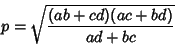(5)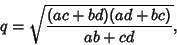(6)

so that. In general, there are three essentially distinct cyclic quadrilaterals (modulo Rotation and Reflection) whose edges are permutations of the lengths,,, and. Of the six corresponding Diagonal lengths, three are distinct. In addition toand, there is therefore a third'' Diagonal which can be denoted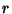. It is given by the equation(7)

This allows the Area formula to be written in the particularly beautiful and simple form(8)

The Diagonals are sometimes also denoted,, and.

The Area of a cyclic quadrilateral is the Maximum possible for any Quadrilateral with the given side lengths. Also, the opposite Angles of a cyclic quadrilateral sum to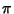Radians (Dunham 1990).

A cyclic quadrilateral with Rational sides,,, and, Diagonalsand, Circumradius, and Areais given by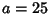,,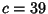,,,,, and.Let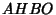be a Quadrilateral such that the anglesandare Right Angles, thenis a cyclic quadrilateral (Dunham 1990). This is a Corollary of the theorem that, in a Right Triangle, the Midpoint of the Hypotenuse is equidistant from the three Vertices. Since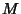is the Midpoint of both Right Triangles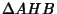and, it is equidistant from all four Vertices, so a Circle centered atmay be drawn through them. This theorem is one of the building blocks of Heron'sderivation of Heron's Formula.Place four equal Circles so that they intersect in a point. The quadrilateralis then a cyclic quadrilateral (Honsberger 1991). For a Convex cyclic quadrilateral, consider the set of Convex cyclic quadrilaterals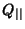whose sides are Parallel to. Then theof maximal Area is the one whose Diagonals are Perpendicular (Gürel 1996).

References

Beyer, W. H. CRC Standard Mathematical Tables, 28th ed. Boca Raton, FL: CRC Press, p. 123, 1987.

Dunham, W. Journey Through Genius: The Great Theorems of Mathematics. New York: Wiley, p. 121, 1990.

Gürel, E. Solution to Problem 1472. Maximal Area of Quadrilaterals.'' Math. Mag. 69, 149, 1996.

Honsberger, R. More Mathematical Morsels. Washington, DC: Math. Assoc. Amer., pp. 36-37, 1991.